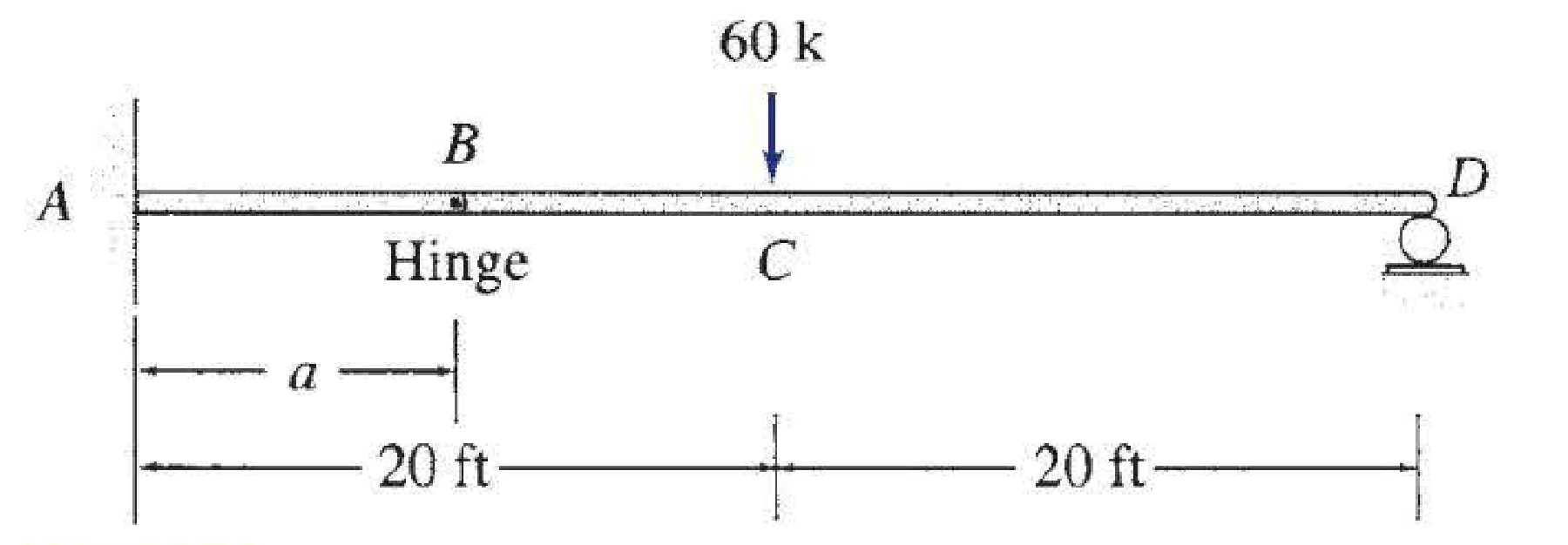# For the beam shown: (a) determine the distance a for which the maximum positive and negative bending moments in the beam are equal; and (b) draw the corresponding shear and bending moment diagrams for the beam.

#### Solutions

Chapter
Section
Chapter 5, Problem 54P
Textbook Problem
137 views

## For the beam shown: (a) determine the distance a for which the maximum positive and negative bending moments in the beam are equal; and (b) draw the corresponding shear and bending moment diagrams for the beam.(a)

To determine

What could be the value of a when the positive and negative bending moments are equal.

### Explanation of Solution

Sign conversion:

Apply the sign convention for calculating the equations of equilibrium as below.

• For the horizontal forces equilibrium condition, take the force acting towards right side as positive (+) and the force acting towards left side as negative ().
• For the vertical forces equilibrium condition, take the upward force as positive (+) and downward force as negative ().
• For moment equilibrium condition, take the clockwise moment as negative and counter clockwise moment as positive.

Apply the following sign convention for calculating the shear and bending moments.

• When the portion of the beam considered is left of the section, then the external force acting to the left are considered as positive.
• When the portion of the beam considered is right of the section, then the external force acting to the right are considered as positive.
• When the portion of the beam considered is left of the section, then the external force acting upward are considered as positive.
• When the portion of the beam considered is right of the section, then the external force acting downward are considered as positive.
• When the portion of the beam considered is left of the section, then the clockwise moments are considered as positive.
• When the portion of the beam considered is right of the section, then the counterclockwise moments are considered as positive.

Calculation:

Show the free-body diagram of the entire beam as in Figure 1.

Consider the section BCD.

Find the vertical reaction at point D by taking moment about the point B.

+MBBCD=060(20a)+Dy(40a)=0Dy=60(20a)(40a) (1)

Consider the entire beam.

Find the moment at point A by taking moment about the point A.

+MA=0MA60(20)+Dy(40)=0MA1200+60(20a)(40a)(40)=0MA=12002400(20a)(40a) (2)

Find the horizontal reaction at point A by resolving the horizontal equilibrium.

+Fx=0Ax=0Ax=0

Find the vertical reaction at point A by resolving the vertical equilibrium.

+Fy=0Ay60+Cy=0Ay60+60(20a)(40a)=0Ay=6060(20a)(40a) (3)

The maximum positive bending moment occurs at the point C

(b)

To determine

Plot the shear force and bending moment diagrams.

### Still sussing out bartleby?

Check out a sample textbook solution.

See a sample solution

#### The Solution to Your Study Problems

Bartleby provides explanations to thousands of textbook problems written by our experts, many with advanced degrees!

Get Started

Find more solutions based on key concepts
SAE 30 oil is contained in a cylinder with inside diameter of 1 in. and length of 1 ft. What is the pressure re...

Engineering Fundamentals: An Introduction to Engineering (MindTap Course List)

Is batch processing still relevant? Why or why not?

Systems Analysis and Design (Shelly Cashman Series) (MindTap Course List)

Explain how P2P networks function, and describe the uses of P2P file sharing.

Enhanced Discovering Computers 2017 (Shelly Cashman Series) (MindTap Course List)

What is a Next Generation Firewall (NextGen or NGFW)?

Principles of Information Security (MindTap Course List)

If a cutting tip sticks in the cutting head, how should it be removed?

Welding: Principles and Applications (MindTap Course List)

If your motherboard supports ECC DDR3 memory, can you substitute non-ECC DDR3 memory?

A+ Guide to Hardware (Standalone Book) (MindTap Course List)